Playing with Familiar Periodic Phenomena: Sound

Sounds will not be available on PDF or HTML versions
Let's begin by "looking" at a familiar periodic phenomena:

We index the notes and write an indexed set of frequency (in Hertz) for each of the notes for one octave above middle-c. We write a function to create a Sound for each note.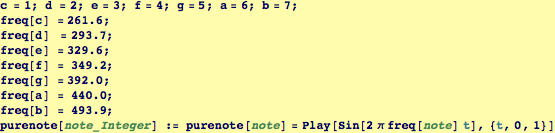We extend the function to get simultaneous notes from a List. We use Thread which takes f[{a,b,c}] to {f[a],f[b],f[c]}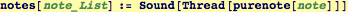Here are examples of their use.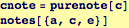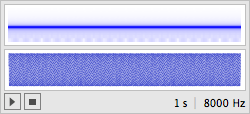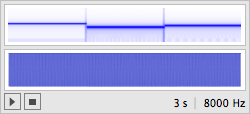We can play with variable amplitudes for a fixed frequency, here we can hear the increased, but non-singular amplitude through zero.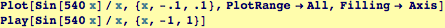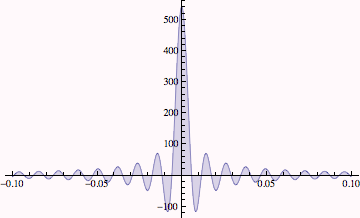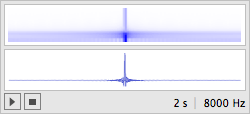We can vary amplitudes and frequencies. Warning, playing with this function can become addictive...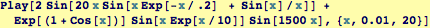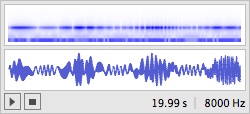Created by Wolfram Mathematica 6.0  (24 October 2007)